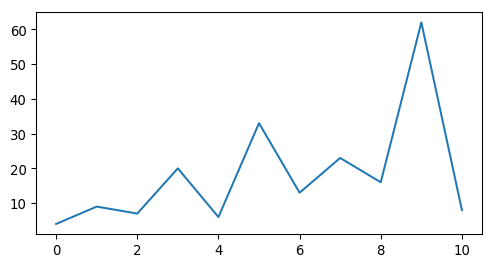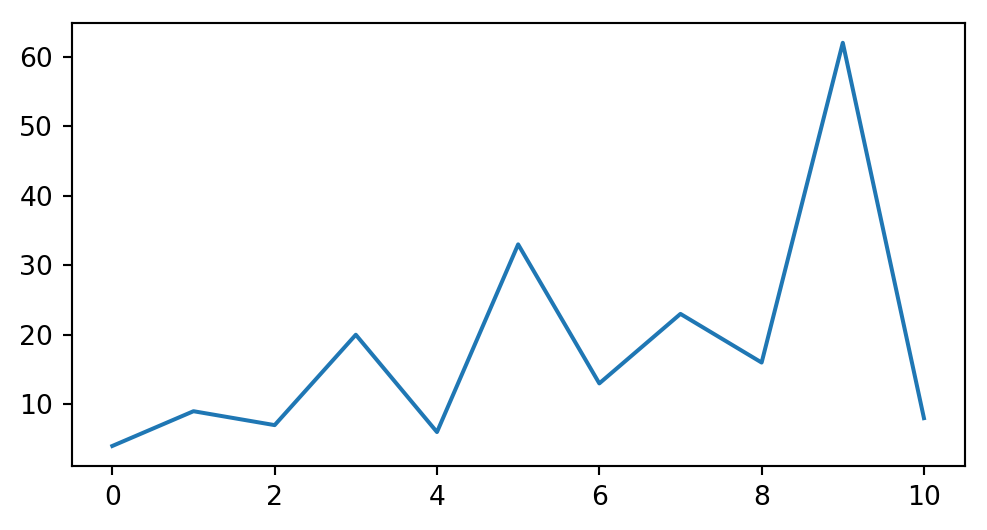# Code Cells¶

## Code, Output, Streams¶

An empty code cell:

:




Two empty lines:

:





:



# 2 empty lines before, 1 after



A simple output:

:

6 * 7

:

42


The standard output stream:

:

print('Hello, world!')

Hello, world!


Normal output + standard output

:

print('Hello, world!')
6 * 7

Hello, world!

:

42


The standard error stream is highlighted and displayed just below the code cell. The standard output stream comes afterwards (with no special highlighting). Finally, the “normal” output is displayed.

:

import sys

print("I'll appear on the standard error stream", file=sys.stderr)
print("I'll appear on the standard output stream")
"I'm the 'normal' output"

I'll appear on the standard output stream

I'll appear on the standard error stream

:

"I'm the 'normal' output"


Note:

Using the IPython kernel, the order is actually mixed up, see https://github.com/ipython/ipykernel/issues/280.

## Cell Magics¶

IPython can handle code in other languages by means of cell magics:

:

%%bash
for i in 1 2 3
do
echo $i done  1 2 3  ## Special Display Formats¶ ### Local Image Files¶ See also SVG support for LaTeX. :  from IPython.display import Image i = Image(filename='images/notebook_icon.png') i  ::  display(i)### Image URLs¶ :  Image(url='https://www.python.org/static/img/python-logo-large.png')  ::  Image(url='https://www.python.org/static/img/python-logo-large.png', embed=True)  ::  Image(url='http://jupyter.org/assets/nav_logo.svg')  :### Math¶ :  from IPython.display import Math eq = Math(r'\int\limits_{-\infty}^\infty f(x) \delta(x - x_0) dx = f(x_0)') eq  : $\displaystyle \int\limits_{-\infty}^\infty f(x) \delta(x - x_0) dx = f(x_0)$:  display(eq) $\displaystyle \int\limits_{-\infty}^\infty f(x) \delta(x - x_0) dx = f(x_0)$:  from IPython.display import Latex Latex(r'This is a \LaTeX{} equation:$a^2 + b^2 = c^2$')  :  This is a \LaTeX{} equation:$a^2 + b^2 = c^2$:  %%latex \begin{equation} \int\limits_{-\infty}^\infty f(x) \delta(x - x_0) dx = f(x_0) \end{equation}  \begin{equation} \int\limits_{-\infty}^\infty f(x) \delta(x - x_0) dx = f(x_0) \end{equation} ### Plots¶ The output formats for Matplotlib plots can be customized. You’ll need separate settings for the Jupyter Notebook application and for nbsphinx. If you want to use SVG images for Matplotlib plots, add this line to your IPython configuration file: c.InlineBackend.figure_formats = {'svg'}  If you want SVG images, but also want nice plots when exporting to LaTeX/PDF, you can select: c.InlineBackend.figure_formats = {'svg', 'pdf'}  If you want to use the default PNG plots or HiDPI plots using 'png2x' (a.k.a. 'retina'), make sure to set this: c.InlineBackend.rc = {'figure.dpi': 96}  This is needed because the default 'figure.dpi' value of 72 is only valid for the Qt Console. If you are planning to store your SVG plots as part of your notebooks, you should also have a look at the 'svg.hashsalt' setting. For more details on these and other settings, have a look at Default Values for Matplotlib’s “inline” Backend. The configuration file ipython_kernel_config.py can be either in the directory where your notebook is located (see the ipython_kernel_config.py in this directory), or in your profile directory (typically ~/.ipython/profile_default/ipython_kernel_config.py). To find out your IPython profile directory, use this command: python3 -m IPython profile locate  A local ipython_kernel_config.py in the notebook directory also works on https://mybinder.org/. Alternatively, you can create a file with those settings in a file named .ipython/profile_default/ipython_kernel_config.py in your repository. To get SVG and PDF plots for nbsphinx, use something like this in your conf.py file: nbsphinx_execute_arguments = [ "--InlineBackend.figure_formats={'svg', 'pdf'}", "--InlineBackend.rc={'figure.dpi': 96}", ]  In the following example, nbsphinx should use an SVG image in the HTML output and a PDF image for LaTeX/PDF output. :  import matplotlib.pyplot as plt  :  fig, ax = plt.subplots(figsize=[6, 3]) ax.plot([4, 9, 7, 20, 6, 33, 13, 23, 16, 62, 8]);Alternatively, the figure format(s) can also be chosen directly in the notebook (which overrides the setting in nbsphinx_execute_arguments and in the IPython configuration): :  %config InlineBackend.figure_formats = ['png']  :  fig  :If you want to use PNG images, but with HiDPI resolution, use the special 'png2x' (a.k.a. 'retina') format (which also looks nice in the LaTeX output): :  %config InlineBackend.figure_formats = ['png2x']  :  fig  :### Pandas Dataframes¶ Pandas dataframes should be displayed as nicely formatted HTML tables (if you are using HTML output). :  import numpy as np import pandas as pd  :  df = pd.DataFrame(np.random.randint(0, 100, size=[5, 4]), columns=['a', 'b', 'c', 'd']) df  :  a b c d 0 81 34 70 12 1 57 58 96 49 2 77 34 61 75 3 70 82 57 54 4 48 75 28 50 For LaTeX output, however, the plain text output is used by default. To get nice LaTeX tables, a few settings have to be changed: :  pd.set_option('display.latex.repr', True)  This is not enabled by default because of Pandas issue #12182. The generated LaTeX tables utilize the booktabs package, so you have to make sure that package is loaded in the preamble with: \usepackage{booktabs}  In order to allow page breaks within tables, you should use: :  pd.set_option('display.latex.longtable', True)  The longtable package is already used by Sphinx, so you don’t have to manually load it in the preamble. Finally, if you want to use LaTeX math expressions in your dataframe, you’ll have to disable escaping: :  pd.set_option('display.latex.escape', False)  The above settings should have no influence on the HTML output, but the LaTeX output should now look nicer: :  df = pd.DataFrame(np.random.randint(0, 100, size=[10, 4]), columns=[r'$\alpha$', r'$\beta$', r'$\gamma$', r'$\delta$']) df  : $\alpha\beta\gamma\delta\$
0 86 59 96 11
1 22 38 37 74
2 80 38 81 67
3 0 44 14 1
4 16 64 45 4
5 87 38 9 14
6 13 95 27 98
7 7 56 9 73
8 21 38 42 64
9 0 1 88 37

:

from IPython.display import YouTubeVideo

:


### Arbitrary JavaScript Output (HTML only)¶

:

%%javascript

var text = document.createTextNode("Hello, I was generated with JavaScript!");
// Content appended to "element" will be visible in the output area:
element.appendChild(text);


Note:

jQuery should be available, but using the readthedocs.org default theme, it’s not. See the issue on Github. Other Sphinx themes are not affected by this.

### Unsupported Output Types¶

If a code cell produces data with an unsupported MIME type, the Jupyter Notebook doesn’t generate any output. nbsphinx, however, shows a warning message.

:

display({
'text/x-python': 'print("Hello, world!")',
'text/x-haskell': 'main = putStrLn "Hello, world!"',
}, raw=True)

Data type cannot be displayed: text/x-python, text/x-haskell

## ANSI Colors¶

The standard output and standard error streams may contain ANSI escape sequences to change the text and background colors.

:

print('BEWARE: \x1b[1;33;41mugly colors\x1b[m!', file=sys.stderr)
print('AB\x1b[43mCD\x1b[35mEF\x1b[1mGH\x1b[4mIJ\x1b[7m'
'KL\x1b[49mMN\x1b[39mOP\x1b[22mQR\x1b[24mST\x1b[27mUV')

ABCDEFGHIJKLMNOPQRSTUV

BEWARE: ugly colors!


The following code showing the 8 basic ANSI colors is based on http://tldp.org/HOWTO/Bash-Prompt-HOWTO/x329.html. Each of the 8 colors has an “intense” variation, which is used for bold text.

:

text = ' XYZ '
formatstring = '\x1b[{}m' + text + '\x1b[m'

print(' ' * 6 + ' ' * len(text) +
''.join('{:^{}}'.format(bg, len(text)) for bg in range(40, 48)))
for fg in range(30, 38):
for bold in False, True:
fg_code = ('1;' if bold else '') + str(fg)
print(' {:>4} '.format(fg_code) + formatstring.format(fg_code) +
''.join(formatstring.format(fg_code + ';' + str(bg))
for bg in range(40, 48)))

            40   41   42   43   44   45   46   47
30  XYZ  XYZ  XYZ  XYZ  XYZ  XYZ  XYZ  XYZ  XYZ
1;30  XYZ  XYZ  XYZ  XYZ  XYZ  XYZ  XYZ  XYZ  XYZ
31  XYZ  XYZ  XYZ  XYZ  XYZ  XYZ  XYZ  XYZ  XYZ
1;31  XYZ  XYZ  XYZ  XYZ  XYZ  XYZ  XYZ  XYZ  XYZ
32  XYZ  XYZ  XYZ  XYZ  XYZ  XYZ  XYZ  XYZ  XYZ
1;32  XYZ  XYZ  XYZ  XYZ  XYZ  XYZ  XYZ  XYZ  XYZ
33  XYZ  XYZ  XYZ  XYZ  XYZ  XYZ  XYZ  XYZ  XYZ
1;33  XYZ  XYZ  XYZ  XYZ  XYZ  XYZ  XYZ  XYZ  XYZ
34  XYZ  XYZ  XYZ  XYZ  XYZ  XYZ  XYZ  XYZ  XYZ
1;34  XYZ  XYZ  XYZ  XYZ  XYZ  XYZ  XYZ  XYZ  XYZ
35  XYZ  XYZ  XYZ  XYZ  XYZ  XYZ  XYZ  XYZ  XYZ
1;35  XYZ  XYZ  XYZ  XYZ  XYZ  XYZ  XYZ  XYZ  XYZ
36  XYZ  XYZ  XYZ  XYZ  XYZ  XYZ  XYZ  XYZ  XYZ
1;36  XYZ  XYZ  XYZ  XYZ  XYZ  XYZ  XYZ  XYZ  XYZ
37  XYZ  XYZ  XYZ  XYZ  XYZ  XYZ  XYZ  XYZ  XYZ
1;37  XYZ  XYZ  XYZ  XYZ  XYZ  XYZ  XYZ  XYZ  XYZ


ANSI also supports a set of 256 indexed colors. The following code showing all of them is based on http://bitmote.com/index.php?post/2012/11/19/Using-ANSI-Color-Codes-to-Colorize-Your-Bash-Prompt-on-Linux.

:

formatstring = '\x1b[38;5;{0};48;5;{0}mX\x1b[1mX\x1b[m'

print('  + ' + ''.join('{:2}'.format(i) for i in range(36)))
print('  0 ' + ''.join(formatstring.format(i) for i in range(16)))
for i in range(7):
i = i * 36 + 16
print('{:3} '.format(i) + ''.join(formatstring.format(i + j)
for j in range(36) if i + j < 256))

  +  0 1 2 3 4 5 6 7 8 91011121314151617181920212223242526272829303132333435
0 XXXXXXXXXXXXXXXXXXXXXXXXXXXXXXXX
16 XXXXXXXXXXXXXXXXXXXXXXXXXXXXXXXXXXXXXXXXXXXXXXXXXXXXXXXXXXXXXXXXXXXXXXXX
52 XXXXXXXXXXXXXXXXXXXXXXXXXXXXXXXXXXXXXXXXXXXXXXXXXXXXXXXXXXXXXXXXXXXXXXXX
88 XXXXXXXXXXXXXXXXXXXXXXXXXXXXXXXXXXXXXXXXXXXXXXXXXXXXXXXXXXXXXXXXXXXXXXXX
124 XXXXXXXXXXXXXXXXXXXXXXXXXXXXXXXXXXXXXXXXXXXXXXXXXXXXXXXXXXXXXXXXXXXXXXXX
160 XXXXXXXXXXXXXXXXXXXXXXXXXXXXXXXXXXXXXXXXXXXXXXXXXXXXXXXXXXXXXXXXXXXXXXXX
196 XXXXXXXXXXXXXXXXXXXXXXXXXXXXXXXXXXXXXXXXXXXXXXXXXXXXXXXXXXXXXXXXXXXXXXXX
232 XXXXXXXXXXXXXXXXXXXXXXXXXXXXXXXXXXXXXXXXXXXXXXXX


You can even use 24-bit RGB colors:

:

start = 255, 0, 0
end = 0, 0, 255
length = 79
out = []

for i in range(length):
rgb = [start[c] + int(i * (end[c] - start[c]) / length) for c in range(3)]
out.append('\x1b['
'38;2;{rgb};{rgb};{rgb};'
'48;2;{rgb};{rgb};{rgb}mX\x1b[m'.format(rgb=rgb))
print(''.join(out))

XXXXXXXXXXXXXXXXXXXXXXXXXXXXXXXXXXXXXXXXXXXXXXXXXXXXXXXXXXXXXXXXXXXXXXXXXXXXXXX